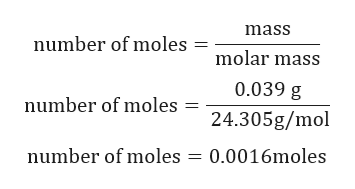# How many moles of hydrogen gas are generated by 0.039 grams of magnesium? Report the answer to four significant figures. DO NOT USE EXPONENTS.

Question
1 views

How many moles of hydrogen gas are generated by 0.039 grams of magnesium? Report the answer to four significant figures. DO NOT USE EXPONENTS.

check_circle

Step 1

Given:

Mass of Magnesium = 0.039 g.

Molar mass of Mg = 24.305 g/mol.

Balanced chemical reaction is given by:

Step 2

Calculation for mo...help_outlineImage Transcriptionclosemass number of moles molar mass 0.039 g number of moles 24.305g/mol number of moles = 0.0016moles fullscreen

### Want to see the full answer?

See Solution

#### Want to see this answer and more?

Solutions are written by subject experts who are available 24/7. Questions are typically answered within 1 hour.*

See Solution
*Response times may vary by subject and question.
Tagged in

### General Chemistry